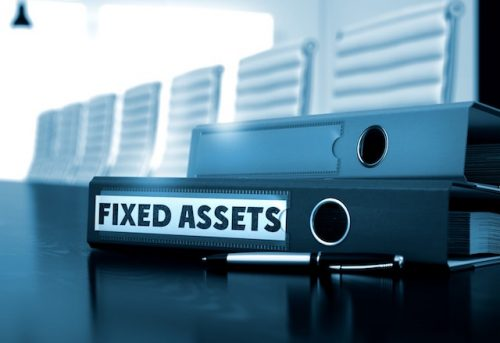# Provisions for the Depreciation of Fixed AssetsWhat is Depreciation?

Depreciation is the reduction in the value of an asset over time. For example, a car purchased for \$10,000 may be worth only \$8,000 one year later because it is not as good as new after a year’s use. The asset has suffered depreciation of \$2,000. This can be calculated by finding the difference between the original value and the reduced value (\$10,000-\$8,000).

Assets may depreciate for a number of reasons:

• Wear and tear: assets become worn out through use.
• Obsolescence: the value of certain assets have decreased because new, more efficient technology has been developed; or machines which were acquired for the production of particular goods are of no further use because the goods are no longer produced.
• Passage of time: an asset acquired for a limited period of time, such as a lease of premises for a given number of years, loses value as time passes. Accountants refer to this as effluxion of time and speak of amortising rather than “depreciating” these assets.

Using up, or exhaustion: mines, quarries and oil wells depreciate as the minerals etc. are extracted from them.

How does depreciation of fixed assets affect accounts?

The accounting treatment of capital expenditure, which is expenditure on fixed assets, is different from the treatment of revenue expenditure. Revenue expenditure is debited to the Profit and Loss Account as it is incurred. Capital expenditure, on the other hand, is on assets intended for use in a business for more than one year, usually for many years. It would be wrong to debit the whole of the cost of a fixed asset to the Profit and Loss Account in the year it was acquired; it would be against the matching principle. Nevertheless, the cost of using fixed assets to earn revenue must be charged in the Profit and Loss Account; that cost is the depreciation suffered in the accounting period.

How to account for depreciation:

There are several methods used to calculate depreciation. The two most common are:

• Straight Line; and
• Reducing Balance

Straight-Line Depreciation:

With this method the total amount of depreciation that an asset will suffer is estimated as the difference between what it cost and the estimated amount that will be received when it is sold or scrapped at the end of its useful life.

The total depreciation is then spread evenly over the number of years of its expected life.

Calculation: (Cost – estimated proceeds on disposal) / estimated useful life in years.

Example: A machine cost \$20,000. It is expected to have a useful life of five years at the end of which time it is expected to be sold for \$5,000 (its residual value). The total depreciation over five years shall be \$(20,000 – 5,000) = \$15,000. The annual depreciation is \$15,000/5 = \$3,000.

Reducing Balance Depreciation:

In this method, depreciation is calculated as a fixed percentage of the stated value of the asset each year, after factoring in the depreciation of the previous year.

Example: A machine costs \$20,000. It is expected to have a useful life of five years. Depreciation is calculated at the rate of 25% per annum on the reducing balance. Calculations for annual depreciation shall be as follows: Year 1 = \$5,000, Year 2 = \$3,750, Year 3 = \$2,813, Year 4 = \$2,109 and Year 5 = \$1,582.

If you are running a small business or managing a large business empire, keeping a tight grip on your finances is of utmost importance. Hiring an accountant or professional bookkeeper who can make financial sense of your business is critical to your success. On average, professional accountant might cost you \$100 to \$200 an hour, however, there are many outsourcing firms that can provide the best accounting services at just a fraction of that cost.